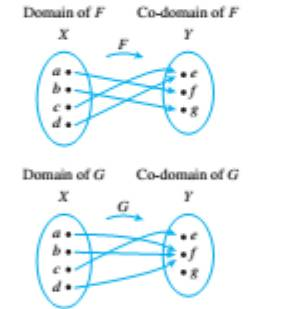Chapter 7.2, Problem 7ES### Discrete Mathematics With Applicat...

5th Edition
EPP + 1 other
ISBN: 9781337694193

#### Solutions

Chapter
Section### Discrete Mathematics With Applicat...

5th Edition
EPP + 1 other
ISBN: 9781337694193
Textbook Problem
1 views

# Let X = { a , b , c , d } and Y = { e , f , g } . Define functions F and G by the arrow diagrams belowIs F one-to-one? Why or why not? Is it onto? Why or why not? Is G one-to-one? Why bot? Is it onto? Why or why not?

To determine

(a)

To check:

Whether the given function F is one-to-one or onto.

Explanation

Given information:

The arrow diagram of given function F is as follows:

Concept used:

A function F is said to be one-to-one function if, and only if, the distinct elements in its domain are mapped with the distinct elements in its co-domain.

A function is said to be onto function if, and only if, each element in its codomain is the image of at least one element in its domain.

Calculation:

F(C)=F(d)=e

Here two element of domain has the same output value.

Therefore this function is not one-to-one function

To determine

(b)

To check:

Whether the given function G is one-to-one or onto.

### Still sussing out bartleby?

Check out a sample textbook solution.

See a sample solution

#### The Solution to Your Study Problems

Bartleby provides explanations to thousands of textbook problems written by our experts, many with advanced degrees!

Get Started